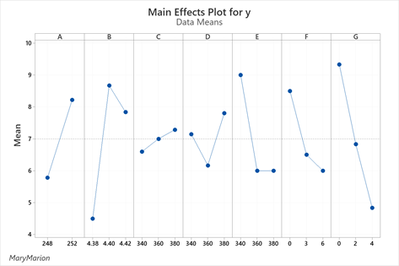## how to panel multiple plots created using proc sgplot?

Here is the basic code:

`proc sgplot data=df;vline G / response=fits stat=mean;run; `

I am doing the same thing for variables A-F. I want to panel them making a main effects mean plot for the response.

Can you help with the syntax? My problem with this is that the variables A-G are continuouos covariates and not levels of a factor.

I think it may be appropriate to use proc sgpanel. Right now I am doing these one at a time using sgplot code.

Formerly, proc gplot was used with proc greplay but I am assuming SAS has upgraded this approach. I am trying to keep this simple.

Thank you.

6 REPLIES 6

## Re: how to panel multiple plots created using proc sgplot?

Data example?

You likely want to add a variable to indicate which "panel" a specific graph belongs in. This might mean restructuring the data, possibly with Proc Transpose so that you have a variable indicating the original variable name. Then use Panelby statement with that new variable.

Likely there is more work involved in reshaping the data then the Sgpanel code.

## Re: how to panel multiple plots created using proc sgplot?

Thank you for the reply. I wanted a verbal response at first. I am having difficulties making a flow diagram of how to prepare the panel plot. I am enclosing a sample data set this time ( modified  on 12-03 9:05am).

## Re: how to panel multiple plots created using proc sgplot?

Can you say more about what you are trying to accomplish?

In your program, you use PROC GENMOD to fit a regression surface that models the response Y as a linear function of the continuous covariates A, B, and C.  Do you want to visualize slices through that surface as a function of each covariate while holding the other covariates fixed at their mean values? If so, use the EFFECTPLOT statement. One choice is as follows:

``````proc genmod data=so;
model y = a b c / dist = normal link=identity lrci obstats;
output out=sonorm pred=fits STDRESDEV=Z;

effectplot fit (x=a);
effectplot fit (x=b);
effectplot fit (x=c);
run; quit;``````

## Re: how to panel multiple plots created using proc sgplot?

In my effort to understand verbal instructions I missed the obvious. Here is a similar graph to what I am doing. Just reduce # of variables down to A B C.## Re: how to panel multiple plots created using proc sgplot?

I need simple means plots that are panelled. Do I want to use sgplot or sgpanel to do it? I was hoping to modify my sgplot code to output a panel. I can transpose like was mentioned. Then A B C become like subjects (rows in dataset)?

## Re: how to panel multiple plots created using proc sgplot?

Here is an updated sas file.

Discussion stats
• 6 replies
• 1202 views
• 0 likes
• 3 in conversation